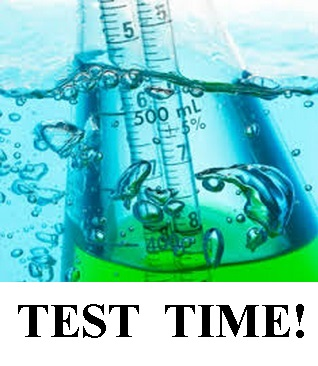# Chemical Reaction Test 2

6 Questions | Attempts: 979
ShareSettingsReactions in Aqueous Solution, Gases, Liquids and Intermolecular Forces

• 1.
The molecular-level representation illustrating solutions of the reactants lead (II) nitrate and potassium iodide is shown above. The reaction equation:Which of the following would be the best molecular level representation after the reaction has taken place?Choose carefully, (A) may not be the first one because of the scrambling of answers)
• A.

A

• B.

B

• C.

C

• D.

D

• 2.
Consider 100.0 mL of a 1.0 M solution of NaCl in a beaker. After several days, you test the solution and find that it has a concentration of 1.33 M. How much water must have evaporation?
• A.

20.0 mL

• B.

25.0 mL

• C.

75.0 mL

• D.

80.0 mL

• 3.
Which of the following statements is true concerning real gases?
• A.

The observed pressure will be less than the ideal pressure, and the volume available for the gas particles is less than the volume of the container.

• B.

The observed pressure will be less than the ideal pressure, and the volume available for the gas particles is greater than the volume of the container.

• C.

The observed pressure will be greater than the ideal pressure, and the volume available for the gas particles is greater than the volume of the container.

• D.

The observed pressure will be greater than the ideal pressure, and the volume available for thee gas particles is less than the volume of the container.

• 4.
A sample of neon gas is contained in the left hand bulb and a sample of helium gas is contained in the right hand bulb of a two bulb container connected by a valve, as shown in the diagram. Initially the valve is closed.The left hand bulb has a volume of 9.00 L, and the Ne gas is at a pressure of 2.00 atm. The right hand bulb has a volume of 3.00L, and the He gas is at a pressure of 4.00 atm. After the valve is opened, what is true about the relative partial pressures of helium and neon? Assume constant temperature.
• A.

The partial pressure of helium is 1.50 times as great as the partial pressure of neon

• B.

The partial pressure of neon is 2.00 times as great as the partial pressure of helium

• C.

The partial pressure of neon is 1.50 times as great as the partial pressure of helium

• D.

The partial pressure of helium is 2.00 times as great as the partial pressure of neon

• 5.
The boiling points of hydrogen chloride and hydrogen bromide are given in the table below. Based on periodicity and intermolecular forces, which of the other two hydrogen halides would have a boiling point that is higher than that of HBr?CompoundHClHBrHiHFBoiling Point (degrees celsius)-85-66??
• A.

HI Only

• B.

HF Only

• C.

HI and HF

• D.

Neither HI nor HF

• 6.
Based on molecular structure and intermolecular forces, which of these substances would you predict to have the greatest viscosity?(Choose carefully)
• A.

A

• B.

B

• C.

C

• D.

D

## Related TopicsBack to top
×

Wait!
Here's an interesting quiz for you.